Reach Us+44-7480-724769
On triple systems and extended Dynkin diagrams of Lie superalgebras 1 | OMICS International
Journal of Generalized Lie Theory and Applications
All submissions of the EM system will be redirected to Online Manuscript Submission System. Authors are requested to submit articles directly to Online Manuscript Submission System of respective journal.

# On triple systems and extended Dynkin diagrams of Lie superalgebras 1

Noriaki KAMIYA1* and Susumu OKUBO2

1Department of Mathematics, University of Aizu, Aizuwakamatsu, 965-8580 Fukushima, Japan E-mail: [email protected]

2Department of Physics, University of Rochester, Rochester, 14627 New York, U.S.A E-mail: [email protected]

*Corresponding Author:
Noriaki KAMIYA
Department of Mathematics, University of Aizu
Aizuwakamatsu, 965-8580 Fukushima, Japan
E-mail: [email protected]

Received date: December 12, 2007; Revised date: March 23, 2008

Visit for more related articles at Journal of Generalized Lie Theory and Applications

#### Abstract

Our aim is to give a characterization of extended Dynkin diagrams of Lie superalgebras by means of concept of triple systems.

#### Preliminaries and examples

Throughout this paper, we shall be concerned with algebras and triple systems over a field Φ that is characteristic not 2 and do not assume that our algebras and triple systems are finite dimensional, unless otherwise specified.

Definition 1.1. For " = ±1 and ± = ±1, a vector space U(ε, δ ) over Φ with the triple product <−,−,−> is called a (ε, δ)-Freudenthal–Kantor triple system if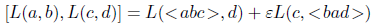where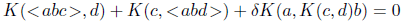Remark 1.1. We note that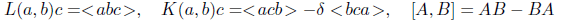are a derivation and an anti-derivation of U(ε, δ), respectively.

Definition 1.2. A (ε, δ)-Freudenthal–Kantor triple system over Φ is said to be balanced if dimΦ {K(x, y)}span = 1

Definition 1.3. For δ = ±1, a triple system over Φ is said to be δ-Lie triple system if the following are satisfied: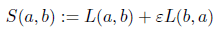For the δ-Lie triple systems associated with (ε, δ)-Freudenthal–Kantor triple systems, we have the following.

Proposition 1.1 (). Let U (ε, δ) be a (ε, δ) Freudenthal–Kantor triple system. If P is a linear transformation of U(ε, δ) such that P < xyz >=< PxPyPz > and P2 = −εδ Id, then (U(ε, δ), [−,−,−]) is a Lie triple system for the case of δ = 1 and an anti-Lie triple system for the case of δ = −1 with respect to the product

[xyz] :=<xPyz> −δ <yPxz> +δ <xPzy> − <yPzx>

Corollary 1.1. Let U(ε, δ) be a (ε, δ)-Freudenthal–Kantor triple system. Then the vector space T(ε,δ) := U(ε,δ)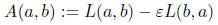U(ε,δ) becomes a Lie triple system for the case of δ = 1 and an anti-Lie triple system for the case of δ = −1 with respect to the triple product defined by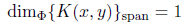¿From these results, it follows that the vector space

L(V ) := Inn Der TT (= L(T, T)T)

where T is a δ-Lie triple system and Inn Der T := {L(X, Y )|X, Y ∈ T}span turns out to be a Lie algebra (δ = 1) or Lie superalgebra (δ = −1) by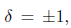Definition 1.4. We denote by L(ε, δ) the Lie algebras or Lie superalgebras obtained from these constructions associated with U(ε, δ) and call these algebras a canonical standard embedding.

Definition 1.5. A (ε, δ)-Freudenthal–Kantor triple system U(ε, δ)) is said to be unitary if the linear span k of the set {K(a, b)|a, b 2 U(ε, δ)} contains the identity endomorphism Id.

Remark 1.2. We note that the balanced property is unitary.

For these standard embedding Lie algebras or superalgebras L(ε, δ), we have the following 5 grading subspaces:

L(ε, δ) = L−2L−1L0L1L2

where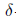#### Lie superalgebras D(2, 1; α), G(3) and F(4)

These constructions of D(2, 1, α), G(3) and F(4) are considered [8, 1]. Briefly describing, we have the following.

1. Let V be a quarternion algebra over the complex numbers. Then V is a balanced (−1,−1) Freudenthal–Kantor triple system with respect to certain triple product and the standard embedding Lie superalgebra L(U) is D(2, 1; α) type’s with dimL(V ) = 17.

2. Let V be a octonion algebra over the complex number. Then V is a balanced (−1,−1) Freudenthal–Kantor triple system with respect to certain triple product and the standard embedding Lie superalgebra L(U) is F(4) type’s with dimL(V ) = 40.

3. Let V be a Im O (= the imaginary part of octonion algebra ). Then V is a balanced (−1,−1)-Freudenthal–Kantor triple system with respect to certain triple product and the standard embedding Lie superalgebra L(U) is G(3) type’s with dimL(V ) = 31.

#### Extended Dynkin diagrams and triple systems

In this section, we will only describe about distinguished extended Dynkin diagram of their canonical Lie superalgebras associated with (−1,−1)-Freudenthal–Kantor triple systems F(4) and G(3) types, because for the other cases we may deal with the explaination by means of the same methods.

(a) For F(4) type distinguished extended Dynkin diagram and usual Dynkin diagram  we have the following: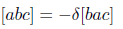U = L−1 = (−1,−1) is a balanced Freudenthal–Kantor triple system with dimU = 8 (cf Sec. 2).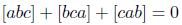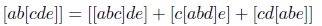L(U) is the standard embedding Lie superalgebra associated with U and dimL(U) = 40, dimL−2 = dimL2 = 1. Then we can easily see its structure as follows: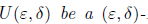and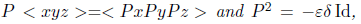= distinguished extended Dynkin diagram with omitted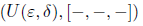of course, L(a, b) = S(a, b) + A(a, b), where S(a, b) is a inner derivation of U, K(a, b) = A(a, b) =<.|.> Id is an anti-derivation of U.

Furthermore, these imply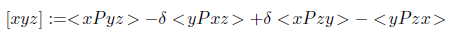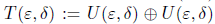Inn DerU = {S(a, b)}span ≅ B3 = Dynkin diagram with omitted(b) For G(3) type distinguished extended Dynkin diagram and usual Dynkin diagram  as well as F(4) we have the following: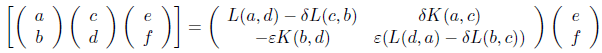U = L−1 = (−1,−1)-balanced Freudenthal–Kantor triple system with dimU = 7 (cf Section 2),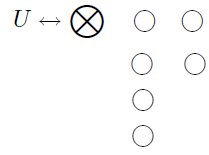L(U) is the standard embedding Lie superalgebra associated with U and dimL(U) = 31, dimL−2 = dimL2 = 1. Then we can easily see its structure as follows: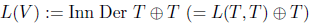(as anti-Lie triple system)

and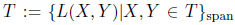= distinguished extended Dynkin diagram with omitted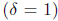Of course, L(a, b) = S(a, b) + A(a, b), where S(a, b) is an inner derivation of U, K(a, b) = A(a, b) =<.|.> Id is an anti-derivation of U. Furthermore, these imply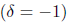#### Acknowledgement

The first author’s research was partially supported by Grant-in-Aid for Scientific Research (No. 15540037 (C), (2)), Japan Society for the Promotion of Science.

#### References

Select your language of interest to view the total content in your interested language

### Article Usage

• Total views: 11734
• [From(publication date):
September-2008 - Nov 15, 2019]
• Breakdown by view type
• HTML page views : 7933Can't read the image? click here to refresh